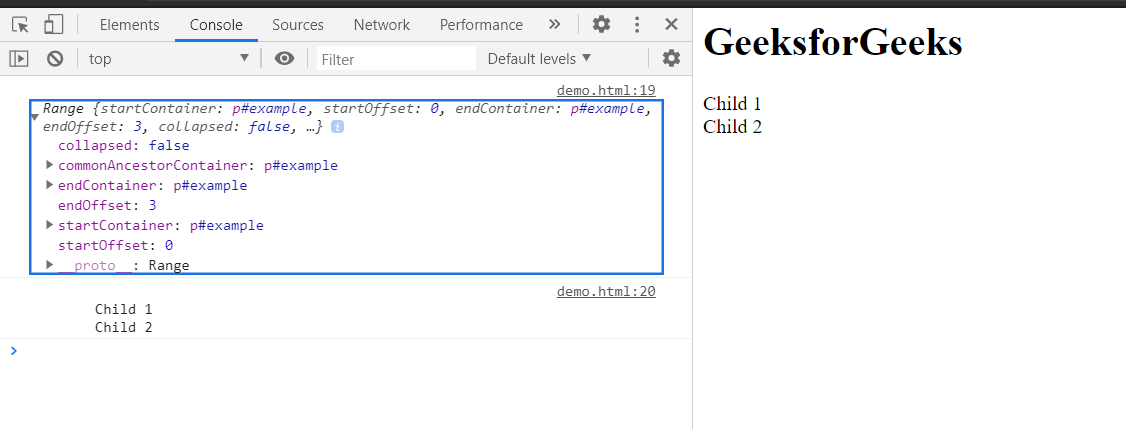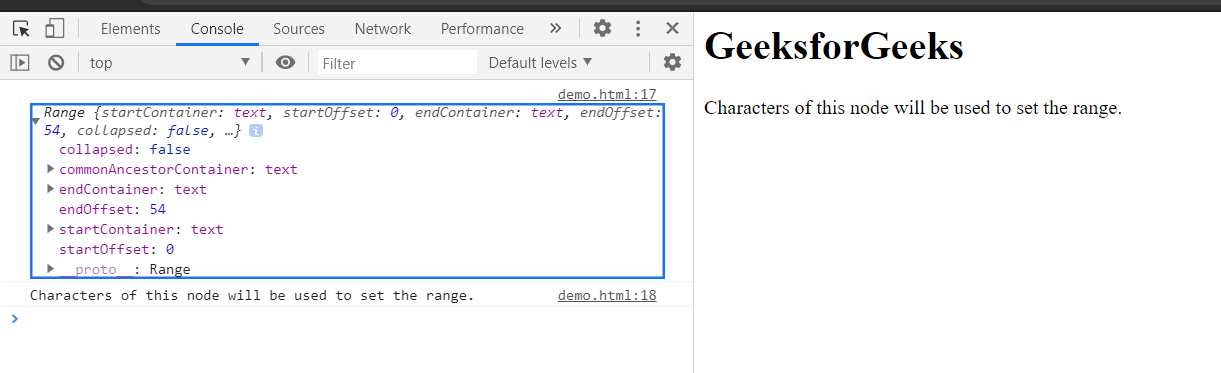# HTML DOM Range setStart() Method

The setStart() method is used to set the starting position of a Range. The startNode can be used as a text node, child nodes etc. The startOffset can be the number of characters from the start of startNode or can be the number of child nodes between the start of the startNode.

Syntax:

`range.setStart(startNode, startOffset);`

Parameters:

• startNode: The Node which is used to start the range.
• startOffset: This parameter is Offset index greater than or equal to zero representing the index for the starting of the Range from the startNode.

Return Value: This method does not return any value.

Example 1: In this example, set the starting of range child nodes of a parent node.

This example uses the setStart() method to set the starting child nodes of the range. Here, we have used setEnd() method to set the ending of the range. For clarity of defined range, we have converted the selected range into text using toString() method.

## HTML

 ` ` `<``html``> ` ` `  `<``body``> ` `    ``<``h1``>GeeksforGeeks ` `    ``<``p` `id``=``"parent"``> ` `        ``Child 1<``br``> ` `        ``Child 2<``br``> ` `    `` ` ` `  `    ``<``script``> ` `        ``const example = document ` `            ``.getElementById('parent'); ` `             `  `        ``const range = document.createRange(); ` `        ``range.setStart(example, 0); ` `        ``range.setEnd(example, 3); ` `        ``console.log(range); ` `        ``console.log(range.toString()); ` `    `` ` ` ` ` `  ` `

Output: In console, the created range can be seen.Example 2: In this example, set the starting of range by getting characters of a text node.

## HTML

 ` ` `<``html``> ` ` `  `<``body``> ` `    ``<``h1``>GeeksforGeeks ` ` `  `    ``<``p` `id``=``"example"``> ` `        ``Characters of this node will ` `        ``be used to set the range. ` `    `` ` ` `  `    ``<``script``> ` `        ``const example = document ` `            ``.getElementById('example'); ` ` `  `        ``const textNode = example.childNodes; ` `        ``const range = document.createRange(); ` ` `  `        ``// Starting of range will  ` `        ``// be 0th character ` `        ``range.setStart(textNode, 0); ` ` `  `        ``// Ending of range will be  ` `        ``// 54th character ` `        ``range.setEnd(textNode, 54); ` `        ``console.log(range); ` `        ``console.log(range.toString()) ` `    `` ` ` ` ` `  ` `

Output: In console, the created range can be seen.Supported Browsers:

• Edge
• Firefox
• Safari
• OperaMy Personal Notes arrow_drop_upCheck out this Author's contributed articles.

If you like GeeksforGeeks and would like to contribute, you can also write an article using contribute.geeksforgeeks.org or mail your article to contribute@geeksforgeeks.org. See your article appearing on the GeeksforGeeks main page and help other Geeks.

Please Improve this article if you find anything incorrect by clicking on the "Improve Article" button below.

Article Tags :
Practice Tags :

Be the First to upvote.

Please write to us at contribute@geeksforgeeks.org to report any issue with the above content.